# 【数据可视化】计算机相关职业信息数据可视化

1、数据获取与清洗。

2、分析计算机相关专业在全国的职位分布。

3、相关计算机专业的任职技术要求。

1、数据获取

① 爬取基本职位信息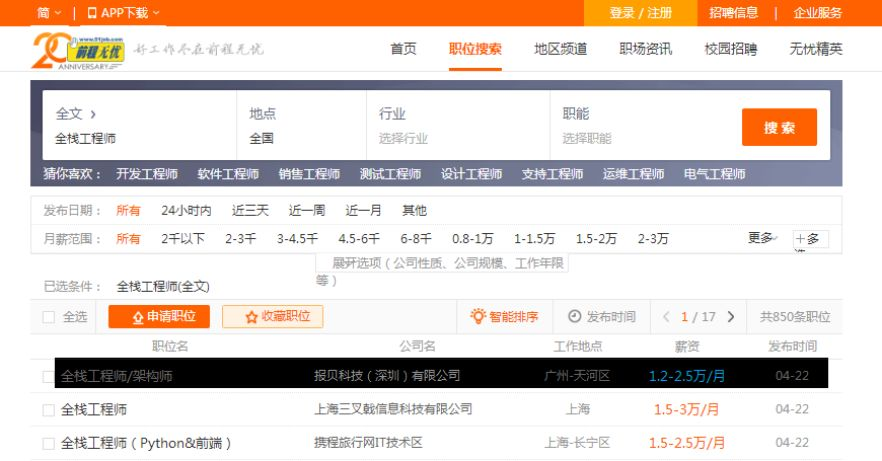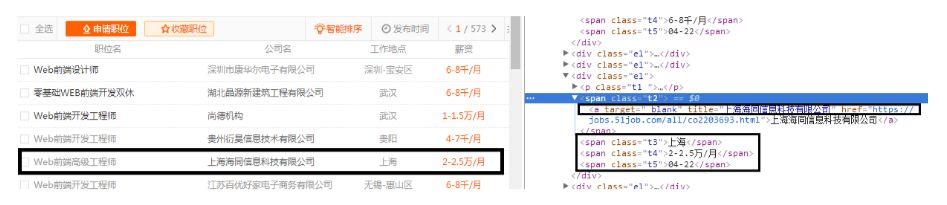reg = re.compile(r'class="t1 ">.*? <a target="_blank" title="(.*?)".*? <span class="t2"><a target="_blank" title="(.*?)".*?<span class="t3">(.*?)</span>.*?<span class="t4">(.*?)</span>.*? <span class="t5">(.*?)</span>',re.S)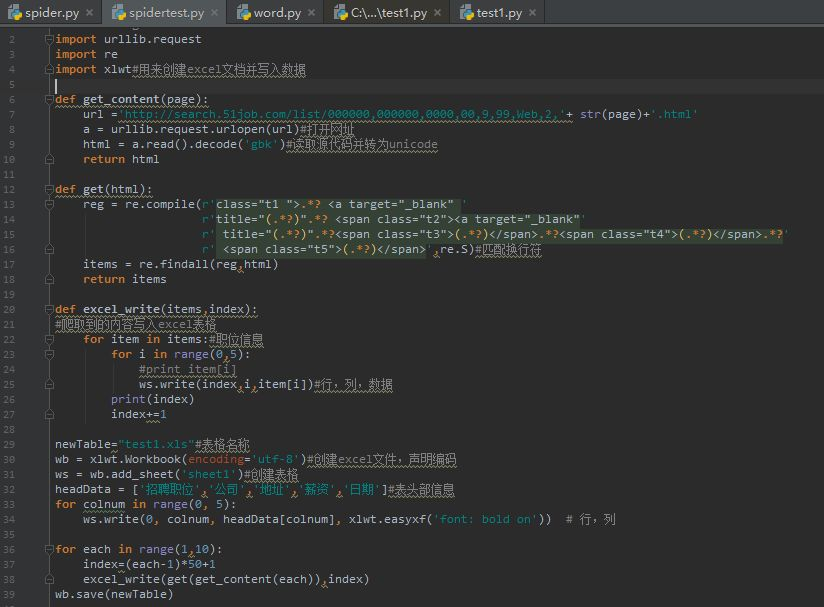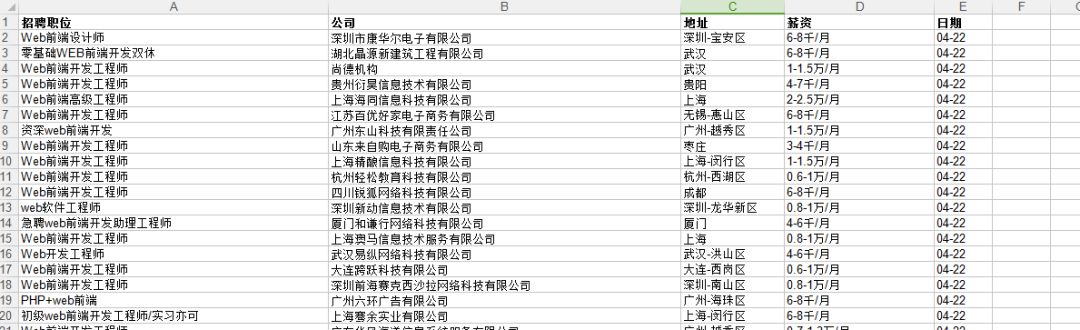② 爬取求职单位关于职位的要求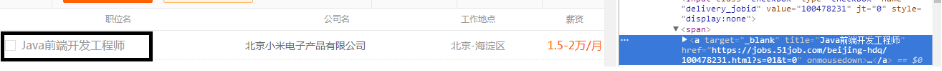first_url = '.....'

response = urllib.request.urlopen(first_url)

html = html.decode('gbk')

#获取子页面的url

content_ul = re.findall(r'<!--列表表格-->(.*?)<!--列表表格 END-->',html,re.S)

#获取包含子页面url的a标签

a_list = re.findall(r'<a target="_blank" .*? onmousedown="">',content_ul)

href_list = []

for a in a_list:

href = re.findall(r'href="(.*?)"',a)

href_list.append(href)

all_article_list =[]

for article_url in href_list:

article_content = re.findall(r'<div class="bmsg job_msg inbox">(.*?) class="mt10"', article_html,re.S)

content_str = ''.join(article_content)

all_article_list.append(content_str)2、数据清洗

① 职位地址分离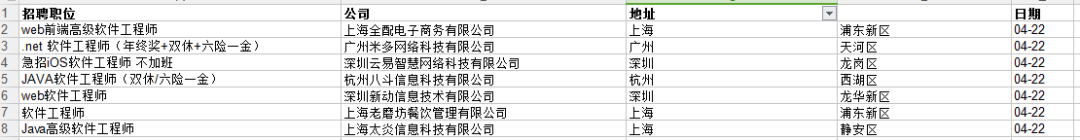2、数据展示

① 工作职位所在地址地图展示

pyecharts模块是一个类似于百度echarts的数据可视化模块。可以很方便地展示出各种美观的信息图。其中最为引人注目的要数地图可视化。其中自带的Geo函数能直接将元素为对象元组的列表显示在地图上包括热力图、点图等等。

web前端工程师分布热力图全部代码如下：import pandas as pd

from pyecharts import Geo

path = r'I:\data\web.csv'

whole = []

for i in range(len(web1)):

a = web1[i],web2[i]

whole.append(a)

geo = Geo("前端工程师全国分布", title_color="#fff", title_pos="center", width=1200, height=600,

background_color='#404a59')

attr, value = geo.cast(whole)

geo.add("", attr, value, type="heatmap", is_visualmap=True, visual_range=[0, 50], visual_text_color='#fff')

geo.show_config()

geo.render()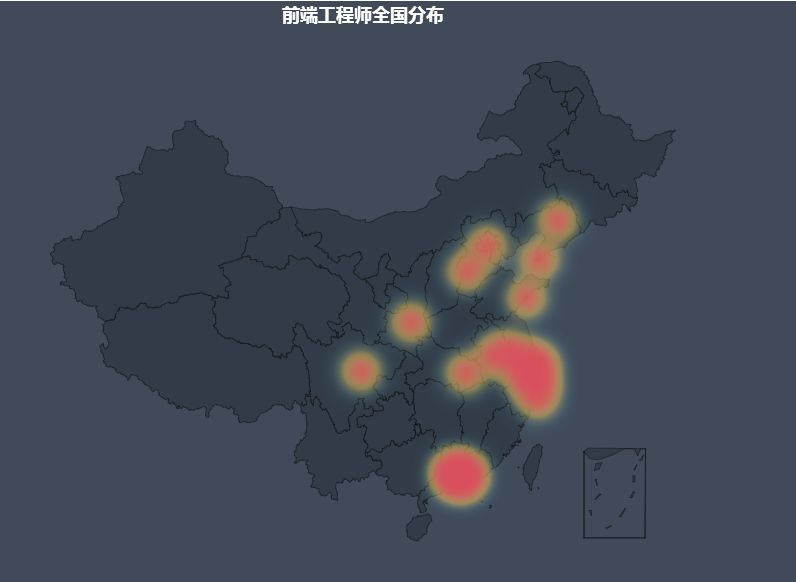② 职位要求词云展示def word_cloud(text=None, img_path=None):

if img_path:

else:

text_from_file_with_apath = text

wordlist_after_jiebe = jieba.cut(text_from_file_with_apath, cut_all=True)

wl_space_split = " ".join(wordlist_after_jiebe)

font = os.path.join(os.path.dirname(__file__), "Diod.ttf")

my_wordcloud = WordCloud(font_path = font,

background_color="white",

stopwords=STOPWORDS,

max_font_size = 40,

).generate(wl_space_split)

plt.imshow(my_wordcloud)

plt.axis("off")

# 绘制词云

plt.figure()

plt.imshow(my_wordcloud.recolor(color_func=image_colors))

plt.axis("off")

plt.figure()

plt.axis("off")

plt.show()

my_wordcloud.to_file(u"wordcloud名称.png")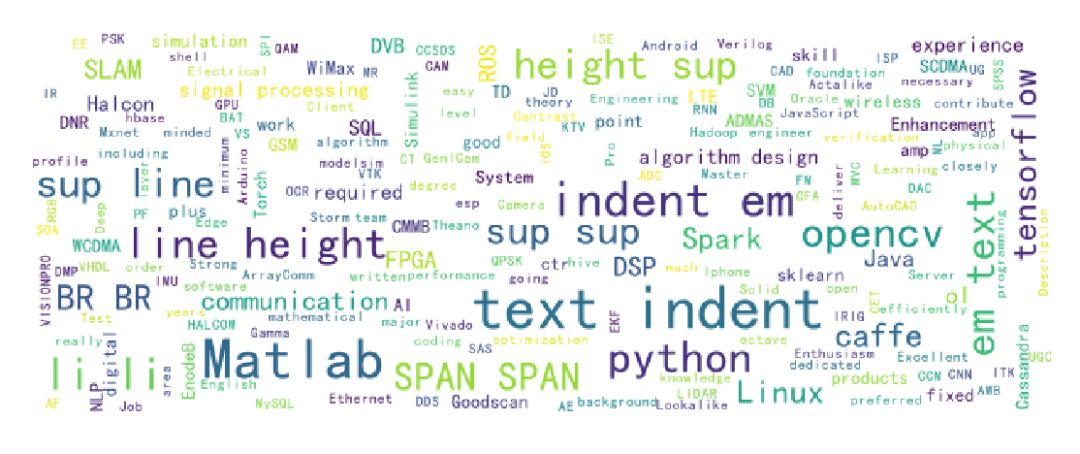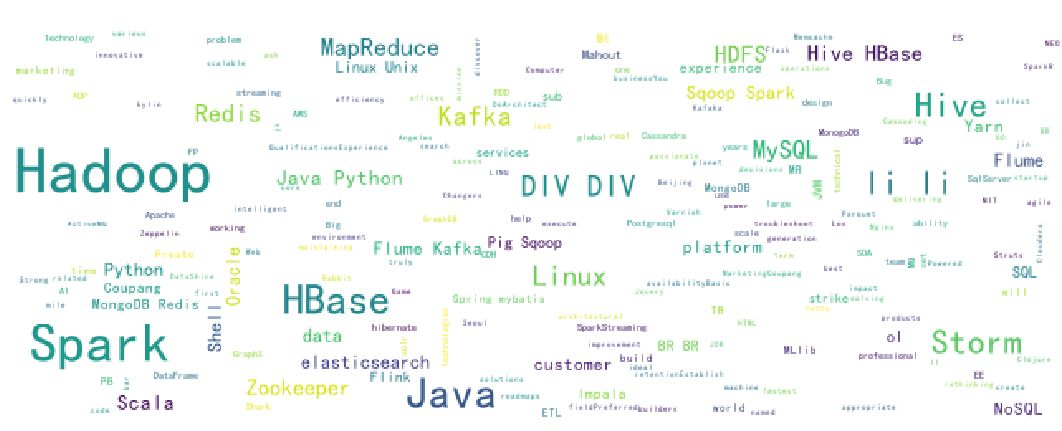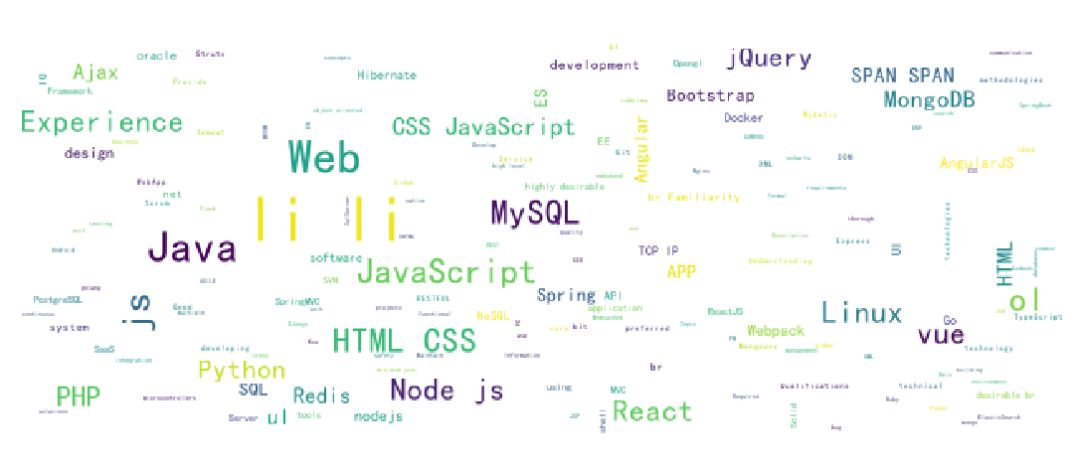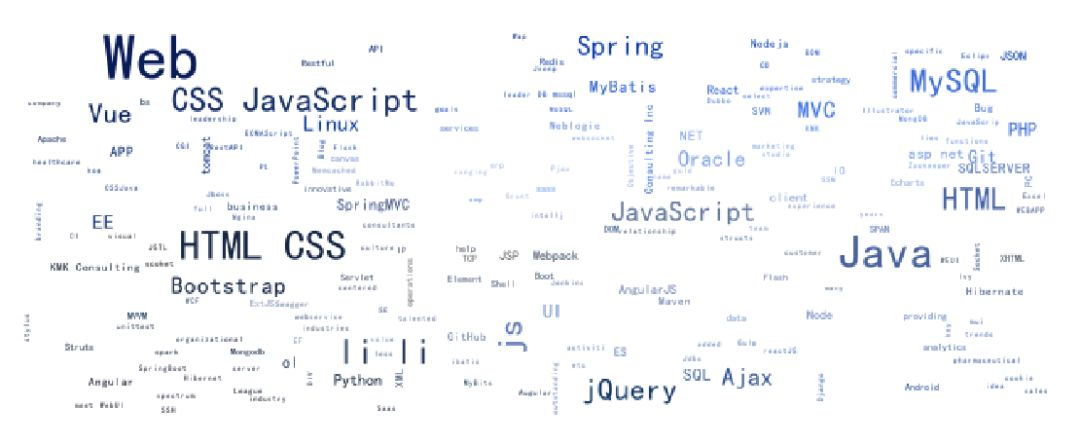dataintellagr@126.com【数据可视化】艾弗森评选名人堂球员原因的可视化分析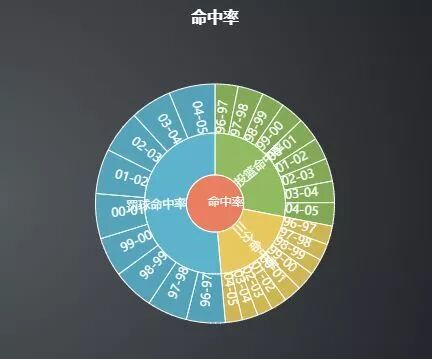【数据可视化】黑龙江经济可视化